Related Articles

# How to Decide Which Excel Lookup Formula to Use?

• Last Updated : 31 Aug, 2021

In Excel, you have a ton of methods to look upon the specific types of values, but the optimal method chosen is what creates a difference. So, what mindset we need to use specific lookup methods in a situation? That’s what we will look at here. But, first, let’s take a look at an example from real life why is it necessary to choose the most optimal approach for lookups.

Example:

Suppose you work at a firm, where lots of revenue sheets(or receipts), and regular employee update sheets come in regularly, but the availability of computers in that region is almost NULL.So, you will use your senses to compute these sheets. Now, you have many approaches, either to read the total of revenues in every sheet column by column, or row by row, or computing small numbers first, and then add or subtract(according to a sheet) big numbers from them, use calculators to compute the sheets, and the ways will be getting more broad, and more in numbers. Now, if you try to compute regular employee sheets, which contain the regular performance of employees along with the daily wage they got, and whether someone left the firm or not. Here also many approaches are possible, as they contain names, numbers, percentages, etc, but the most efficient way to be picked is most crucial.

So, in Excel also, you need to pick the most suitable way to lookup the values in Excel. But first, let’s take a look at what ways we have to lookup values in Excel.

1. VLOOKUP
2. HLOOKUP
3. INDEX MATCH
4. VLOOKUP MATCH
5. OFFSET MATCH

Now, lets look at them briefly:

### 1.VLOOKUP

Vlookup, as the name suggests,is looking the data in vertical manner,i.e it will look data in column(s). Here, capital V signifies the vertical lookup of data. This method is the classical method for performing lookups. Its sole purpose is to return the value by taking the reference of the first column of the table. Its usage is quite simple, and the syntax for using this method will be:

```VLOOKUP (the value to be searched, range of table onto which values will be searched,
Numerical value of column from which data will be retrieved,
LOOKUP RANGE=TRUE/FALSE(OPTIONAL))```

Now, we will get better understanding of syntax when we will see this applied on a dataset. So, an example dataset here will be the employee name, along with their revenue generated per day, along with their daily wage.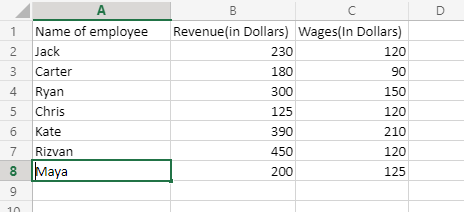Now,you want to find out how much did Kate earned today, so we will use VLOOKUP formula to fetch the value. So,the formula will be:

`=Vlookup(Kate;A2:C:8;2;FALSE)`

Now, we will understand why we passed these values in the arguments’ step by step:

1. We passed Kate as our first argument, as the revenue of Kate was to be fetched, and VLOOKUP always takes the reference of the first column of dataset.
2. The range of values that will be traversed to fetch value is A2 to C8,i.e in short we are traversing the whole dataset, as we gave the coordinate of starting cell of dataset(excluding column name), and the last value of dataset,i.e C8.
3. The column that was storing the revenue of employees was the 2nd column, so 2 was passed in the 3rd argument.
4. Now, this lookup range has been set as false, as we don’t want values to be searched approximately, we want precise value, so the last argument is set as FALSE. In cases, when you want the output to be near exact we set this argument as TRUE.

The important point to note about VLOOKUP is that it only knows how to look up data in fashion from left to right only.

The major disadvantage of VLOOKUP is that it is not compatible with the dynamic world, as our datasets will change on a day-to-day basis. So, to enhance VLOOKUP, we use VLOOKUP with other functions.

### 2.HLOOKUP:

Just as V in VLOOKUP means vertical lookup in data, H in HLOOKUP means horizontal lookup in data. Its main purpose is to return the desired value by looking in a horizontal fashion,i.e in rows by taking the reference of the numerical value of the first row. Its syntax is quite familiar to VLOOKUP’s syntax.

```=HLOOKUP (the value to be searched,range of table onto which values will be searched,
index of row onto which value will be searched,
LOOKUP RANGE=TRUE/FALSE(OPTIONAL))```

Now, considering a dataset, where we are keeping track of how many copies of action movies were sold on consecutive days,so the dataset will be: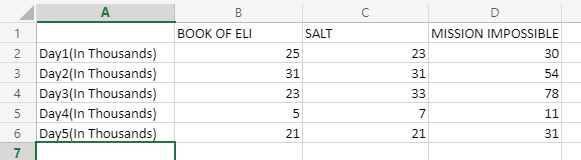Now, your task is to find how many copies of “SALT” were sold on Day2. So here you can see that the data searching shifted from vertical to horizontal. Now,the formula will be:

`=HLOOKUP(SALT;B1:D6;2;FALSE)`

This will fetch the following output–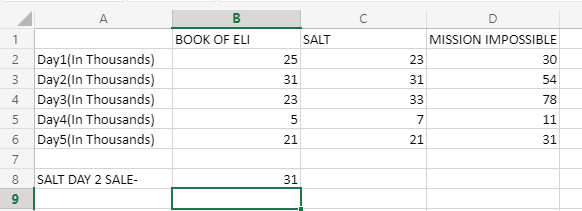Now, how this formula worked? Lets see it by looking at what arguments are getting passed in the HLOOKUP function:

1. The first argument was name of movie(or attribute) whose value has to be fetched.
2. The second argument takes the range of tables on which value will be searched.
3. The third argument will contain the number of rows, on which value will be searched, and in this case, we want day2’s sale, and that is in row 2(keeping in mind we started with B1 as our starting row, so this row will be counted as row 2),so 2 is passed here.
4. The fourth argument is functioning same as in VLOOKUP’s fourth argument,i.e to be exact, we passed FALSE, and you can also pass boolean value of FALSE i.e 0 here.

HLOOKUP is not very frequently used, as its requirements say that, you should be placing the search value in the top row of the database.so, just like VLOOKUP, HLOOKUP alone is not compatible with current settings, so instead we use HLOOKUP with different methods.

### 3.INDEX MATCH:

Index match utilizes two main properties,i.e “INDEX” and “MATCH”. These two, when combined, can make the searching(lookup) criteria more flexible,i.e no more restrictions, that you can use this method for vertical lookups, this method for horizontal lookups, etc INDEX MATCH removes this thing, and make the lookup process more flexible.

Now, to understand this method, we will look at two examples each one belonging to INDEX and MATCH.

INDEX Function: The INDEX function,is pretty basic,and its function is to return the value in that cell,whose value is specified in the function’s arguments.So.lets take a look at its syntax–

```INDEX(Range of table you want to search the value;
numerical value of row on which value is to be searched;
numerical value of column on which value is to be searched)```

Now, let’s look at above dataset only ,and try to understand, how INDEX works. Suppose you want to know, that how much did “Book of eli” earned on DAY3. Now, you know that day 3 entries are done on 3rd row, and book of eli’s entries are done on 2nd column, so the formula will look like:

`=INDEX(A2:D6;3;2)`

Now, the output will look like: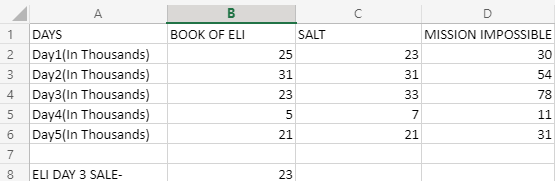Now, how this formula worked? Let’s take a look at what we passed in arguments in INDEX function–

• A2:D6: This will tell INDEX where to search in dataset.
• 3: The row number on which value is to be searched(keeping in mind, we started with A2,so in that context, the row number becomes 3)
• 2: The column number on which value is to be searched.

If you are familiar with basic concept of coordinate geometry, you should have noticed that it returned us the value stored at (3,2) position.

Now, moving onto match function,

MATCH Function: The MATCH function will tell us what is the position of an item, which will be passed as the first argument of this function, and it is independent of searching in the horizontal manner or vertical manner. This function is very powerful in finding values in very large datasets. Now let’s take a look at an example of the MATCH function.

Considering a dataset, where there are student’s names, and one student has left the school, and the student’s name is “RAJ MEHRA”. So, we want to find out where he is located on this dataset. The dataset used will be: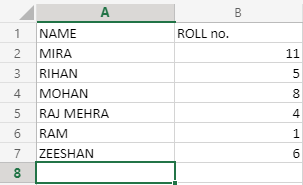Now, we will apply our match function on column name “NAME”, and the formula will look like this:

`MATCH("RAJ MEHRA";A2:A7;0)`

This will output 4 as our answer, as “RAJ MEHRA” is on the 4th row. Now, how this formula worked? Let’s take a look at its arguments:

1. ” RAJ MEHRA “: This argument accepts a string, that will be searched in the given range of searching.
2. A2:A7: The range on which value will be searched.
3. 0: This argument is the same as we saw in VLOOKUP OR HLOOKUP, and here we set this argument as 0 or FALSE, which means we want an exact matching behavior with the string we passed. This argument, when passed as 1 or TRUE, will go for approximation for matching with the string passed.

Now, as we understood INDEX, MATCH individually, now we will look at INDEX, MATCH in action mutually,i.e how they work together.

Considering the expanded version of the dataset we created in the above example, now we will add the area in which the students are studying in,i.e in which departments of study they are in. So, the dataset will be: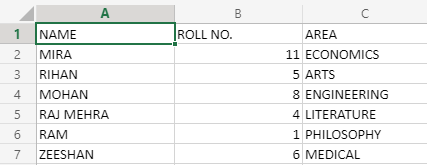Now, the thing is, suppose you don’t know on what row is Mohan’s data stored, but you want to fetch the area of study of MOHAN, so here MATCH function will help the INDEX FUNCTION to locate the row number on which value is stored, by taking the name of the student in its first argument. The match function will get passed as the second argument in the INDEX function, as we are not aware of the row number. So, the formula of this will look like:

`=INDEX(B2:C7;MATCH("MOHAN";A2:A7;0);3)`

This formula is utilizing both index and match, which makes this formula very efficient.

The only disadvantage it holds is that it is not compatible with very large datasets.

### 4.VLOOKUP MATCH:

As the name suggests, this duo will enhance the searching(lookup) capability of the VLOOKUP method. What VLOOKUP was doing is that it was searching in only a vertical manner,i.e down the column range only. But, as we all know this will not fulfill the requirements of searching in the real world. Now, using this method, we can search in 2D form,i.e in a matrix, rather than just going up to down and following a 1D method. As we have understood both VLOOKUP and MATCH in separate, so let’s understand this method directly from an example:Now, considering the same dataset as created in the above example, we want to know the ROLL NO. of Rihan. So, we will use VLOOKUP method to do this. The formula for this will be:

`=VLOOKUP("RIHAN";A1:C7;MATCH(B1,A1:C1))`

This will return 5 as our answer. But, as you can see we used VLOOKUP and found out the value which is contained in some other column’s cell. So, here you can see the expansion of VLOOKUP. Now, how did this formula work? Let’s see it argument by argument.

1. “RIHAN”: This will be the reference, that VLOOKUP will take to search its value.
2. “A1:C7”: the ranges on which value will be searched.
3. “MATCH(B1,A1:C1)”: This part makes vlookup more flexible. Here if we look at what match function will return, so it will return 2,as roll numbers are contained in 2nd column, Now, the array range on which column will be searched, is going to be A1 to C1,i.e column name contained row.

Now, as soon as VLOOKUP reaches the cell where “RIHAN” is located, the match function will direct the control to the second column, and here you can see VLOOKUP changes itself from 1D searching to 2D searching.

### 5.OFFSET MATCH:

To understand this concept, we need to understand what offset is.

OFFSET Method: This method is used mostly in dynamic searching or shall we say to reach or to point to some specific cell, by starting from any cell. i.e to take reference of this cell. And to reach some other cell. The only requirement for offset to work is that your dataset should be a 2d matrix, not just one cell, or a linear dataset. Now, let’s take a look at its syntax:

`OFFSET(Starting cell;row offset;column offset;height for rows;height for columns)`

Now, let’s take a look at how its parameters are working through an example. For this, you should have a good image of how rows and columns are traversed in a matrix. You can use an empty dataset for reference.

Suppose you are standing on cell B2, and want to reach cell E6, so here you can see that our starting cell is B2. Our row offset,i.e how many rows far is our targeted cell is, so in this case, our row offset is 4.Our column offset,i.e how many columns far are our targeted cell located, so in this case, our column offset is 3.So our offset formula will look like–

`=OFFSET(B2;4;3)`

Now, we can also reference a range of values in our dataset using only one cell, and in this, we will utilize our last two parameters also.

Suppose you want to reference C2:C6, starting from B2 only, so in this row offset will be 0, as starting cell of the range, ie C2, is in the same row, as in B2.The column offset will be 1, as the C2 cell is one column ahead of the B2 cell. Height for rows, will be the number of cells contained in the range given, so in C2 to C6, the range 5 cells are covered, so height for rows will take 5 as its argument. For column height, as the name suggests, it is referring to how many cells in a horizontal manner are given a range of cells covering. As we can see the columns are going in a vertical manner, so our column height is 1.To be more precise, height for columns is no other than the width of columns your range of values is covering. So the formula for this will be:

`=OFFSET(B2;0;1;5;1)`

In Excel, it is not advised to put height for rows and height for column’s negative values.

Now, we will see an offset, match working together: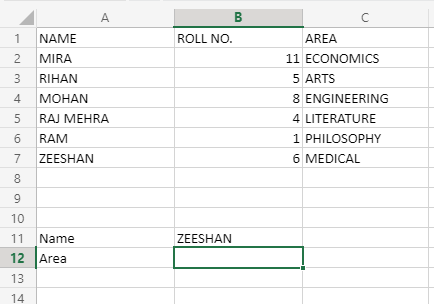Considering this dataset, we want to know in which area of study is Zeeshan studying. So, first, let’s see how the syntax of offset match looks like:

```=OFFSET(Reference cell,MATCH(RowLookupNumber,RowLookupRange,Boolean for matching precision),
MATCH(ColumnLookupNumber,ColumnLookupRange,Boolean for matching precision))```

To understand this syntax, let’s consider the above situation and try to find out the area of study in which Zeeshan studies using offset match. So, the formula will be:

`=OFFSET(A1;MATCH("ZEESHAN";A2:A7;0);MATCH("AREA";A1:C1;0))`

Putting this formula in B12 cell, the answer will be MEDICAL.Now, how does this work? Let’s understand it argument by argument:

1. A1: This is the reference from which offset will start itself for searching.
2. “ZEESHAN”: This is the lookup value that match will search in the given range.
3. A2:A7: This is the range for row lookup value, in this range match will search for the keyword “ZEESHAN”.
4. 0: This argument will signify for the precise searching, which means 0, and for approximate searching,1 is passed here.
5. “AREA”: This is the column lookup value that match will find in the given range. So, here you can see, offset’s parameters are getting directed by the match’s return values to go in 2D form searching.
6. A1:C1: This is the column lookup range, that will be used by match to search for the column named “AREA”.
7. 0: This argument will signify for the precise searching, which means 0, and for approximate searching,1 is passed here.

The major advantage this method holds, other than other methods, is that it is a much faster and less time-consuming search process in Excel.

Now, we will see that how we can compare two tables:

### Comparison of Tables: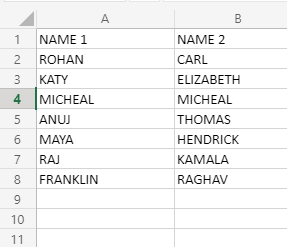Now, let’s suppose we have 2 tables, name 1 and name2, and these represent employees from 2 different departments, so their name obviously can’t be the same. So, we will compare the 2 tables using our concept of comparison of tables.

So, here we will use a very basic IF formula to do the above task. It works like an “if” conditional statement, which is in most programming languages. So, the formula for doing the above task will be–

`=IF(A2=B2;"VERIFY";"CLEAR")`

Now. when we will apply this formula in cells C2 to C8, the resulting dataset will look like this: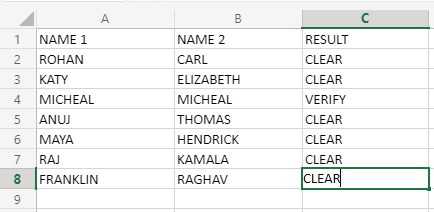Now, working of this formula, as it is just comparing the 2 values in corresponding cells of columns A and B, and returning CLEAR, if the values are not matching, otherwise, it will return VERIFY, as returned by comparing A4 WITH B4. This process can also be done, without using the IF formula, and simply just comparing corresponding cells of A and B. This will return a traditional TRUE value if the match is found, otherwise FALSE would be returned. So, the IF formula is only used when we want to fabricate our return values.

Attention reader! Don’t stop learning now. If you are an Excel beginner (or an intermediate) and want to learn Excel, Geeksforgeeks brings the perfect course for you to start, Diving Into Excel

My Personal Notes arrow_drop_up